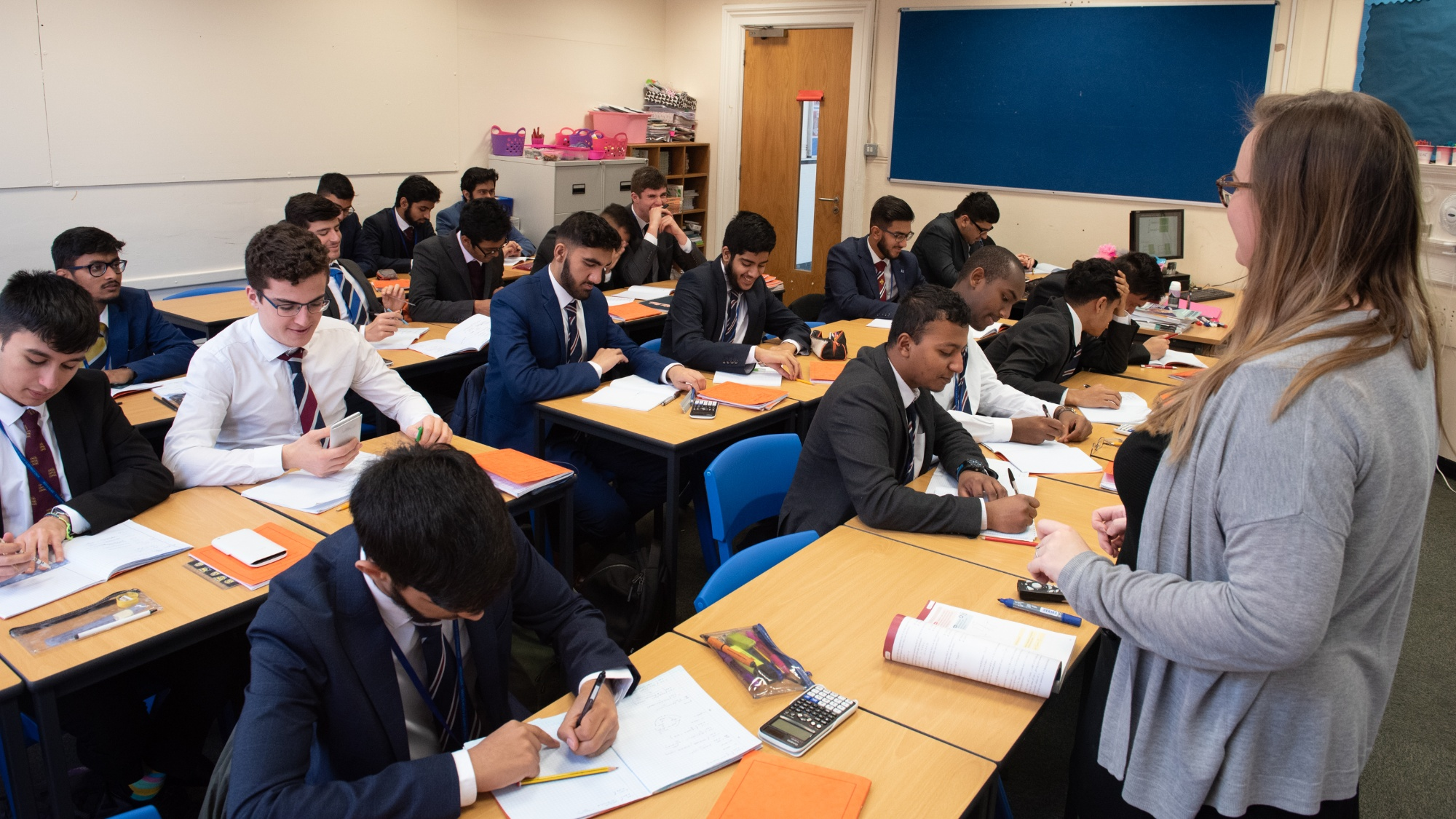•# Further Mathematics

In year 12, further mathematics is taught in parallel with the A Level Mathematics course. New topics are introduced such as complex numbers and matrices. Strands of mathematics taught are categorised into Core Pure and Applied (Applied consists of Further Statistics and Further Mechanics). The curriculum promotes analytical and evaluative skills which are essential to modelling mathematical problems. Students are required to solve varying types of problems both in class and independently, notably from the Pearson Core Pure 1 and FS1/FM1 textbooks.

Pure

• Complex numbers
• Argand diagrams
• Series
• Roots of polynomials
• Volumes of revolution
• Matrices
• Linear transformations
• Proof by induction
• Vectors

Applied

• Momentum and impulse
• Work, energy and power
• Elastic collisions in one dimension
• Discrete random variables
• Poisson distribution
• Hypothesis testing
• Chi-squared tests

In year 13 we build upon the mathematical methods and techniques learnt in year 12. We continue to study topics from the strands of Core Pure, Further Mechanics and Further Statistics allowing all students to continue to grow in confidence and competence. Students complete work from a variety of sources,  notably the Pearson Core Pure 1 and FS1/FM1 textbooks.

Core Pure

• Complex numbers
• Series
• Methods in calculus
• Volumes of revolution
• Polar coordinates
• Hyperbolic functions
• Methods in differential equations
• Modelling with differential equations

Applied

• Momentum as a vector
• Elastic strings and springs
• Elastic collisions in two dimensions
• Geometric distribution
• Negative binomial distribution
• Hypothesis testing
• Central limit theorem
• Chi-squared tests
• Probability generating functions
• Quality of tests